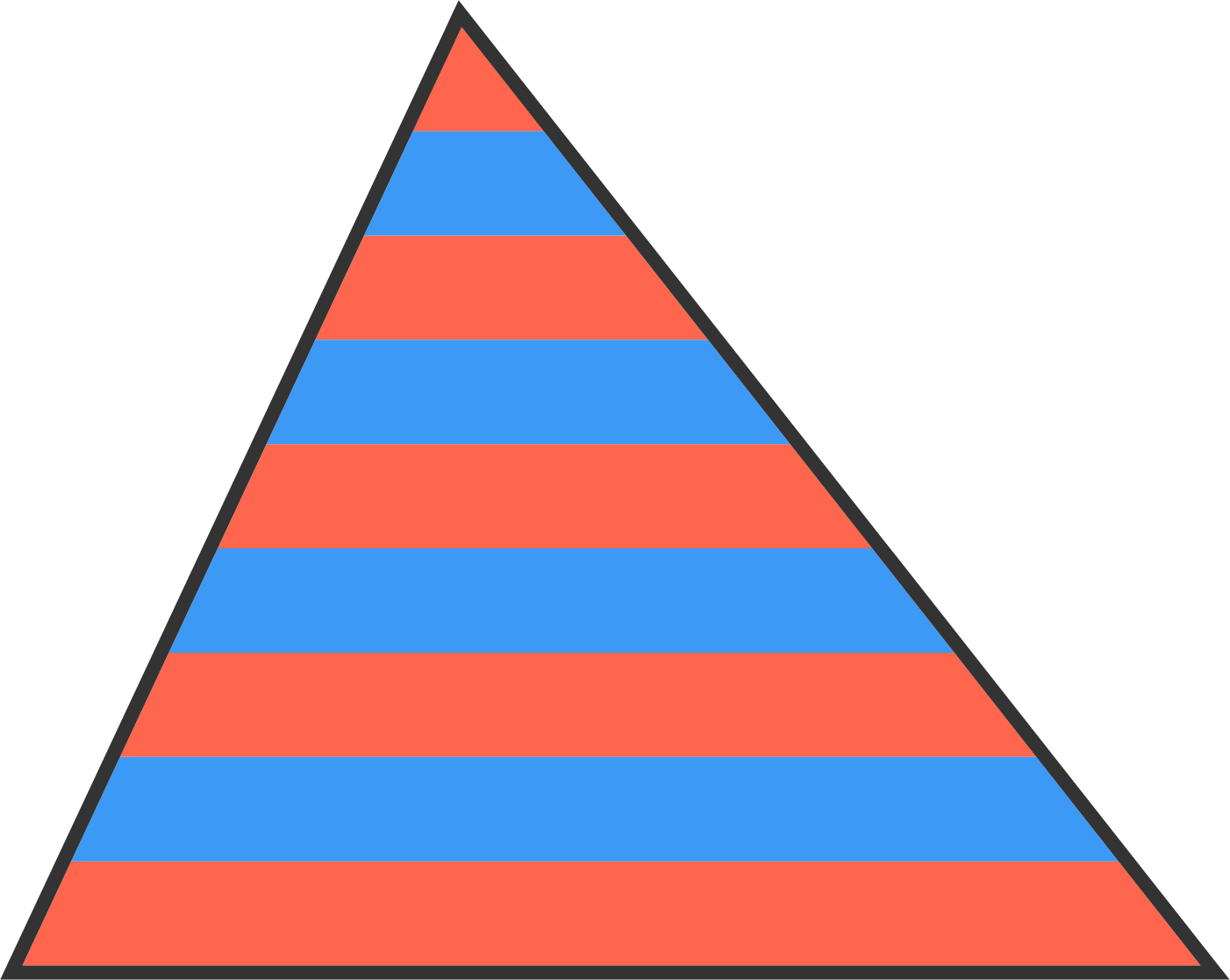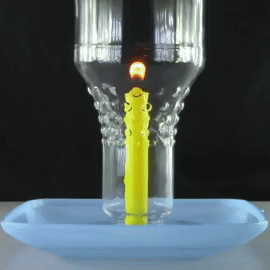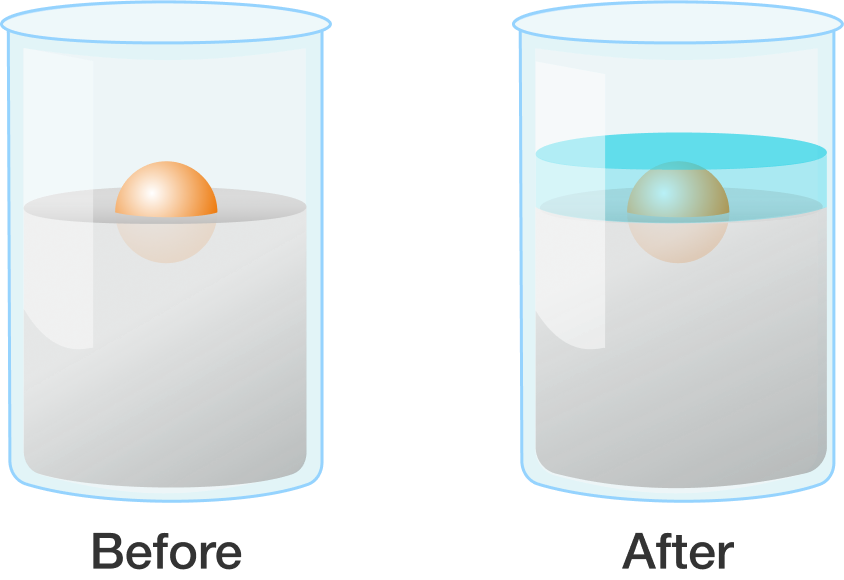# Problems of the Week

Contribute a problem

# 2018-08-06 BasicThis triangle is divided into nine parallel stripes of equal height. The total area of the red stripes is 145.

What is the total area of the blue stripes?

If $1 + 3 + 5 + \cdots + 99 = 2500 ,$ then what is $3 + 5 + 7 + \cdots + 101 ?$

$a$ and $b$ are real numbers, and $a\div b=a\times b=a+b.$

What is $a-b?$A bottle is placed over a lit candle sitting in a bowl of water.

The amount of oxygen the candle can use is more than the $\ce{CO_2}$ it produces, so water is sucked up into the bottle from the bowl, making the water level inside the bottle slowly rise.

What will happen to this water level when there is no more oxygen left in the bottle and the candle is extinguished?

Before, a ball was floating in liquid mercury. After, water has been poured on the ball until the ball is entirely submerged.

How has the ball's position changed?×